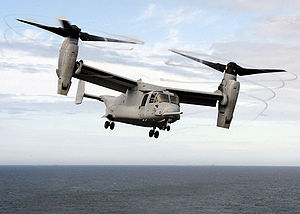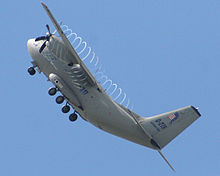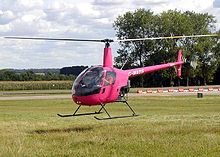The MV-22 Osprey tiltrotor has a relatively high disk loading, producing visible blade tip vortices from condensation of the marine air in this photo of a vertical takeoff.C-27J Spartan with propeller tip vortices condensation. The C-27J uses the same engines as the MV-22, but has higher disk loading.Piston-powered light utility helicopters like this Robinson R-22 have relatively low main rotor disk loading

## Rotors

Disc loading of a hovering helicopter is the ratio of its weight to the total main rotor disc area. It is determined by dividing the total helicopter weight by the rotor disc area, which is the area swept by the blades of a rotor. Disc area can be found by using the span of one rotor blade as the radius of a circle and then determining the area the blades encompass during a complete rotation. As the helicopter is maneuvered, disc loading changes. The higher the loading, the more power needed to maintain rotor speed.

Increasing the weight of a helicopter increases disk loading. For a given weight, a helicopter with shorter rotors will have higher disk loading, and will require more engine power to hover. A low disk loading improves autorotation performance in rotorcraft. Typically, an autogyro (or gyroplane) has a lower rotor disc loading than a helicopter, which provides a slower rate of descent in autorotation.

## Propellers

In reciprocating and propeller engines, disk loading can be defined as the ratio between propeller-induced velocity and freestream velocity.[citation needed] Lower disk loading will increase efficiency, so it is generally desirable to have larger propellers from an efficiency standpoint. Maximum efficiency is reduced as disk loading is increased due to the rotating slipstream; using contra-rotating propellers can alleviate this problem allowing high maximum efficiency even at relatively high disc loadings.

The Airbus A400M fixed-wing aircraft will have a very high disk loading on its propellers, and this had to be factored into the design.

## Theory

The momentum theory or disk actuator theory describes a mathematical model of an ideal actuator disk, developed by W.J.M. Rankine (1865), Alfred George Greenhill (1888) and R.E. Froude (1889). The helicopter rotor is modeled as an infinitely thin disc with an infinite number of blades that induce a constant pressure jump over the disk area and along the axis of rotation. For a helicopter that is hovering, the aerodynamic force is vertical and exactly balances the helicopter weight, with no lateral force.

The upward action on the helicopter results in a downward reaction on the air flowing through the rotor. The downward reaction produces a downward velocity on the air, increasing its kinetic energy. This energy transfer from the rotor to the air is the induced power loss of the rotary wing, which is analogous to the lift-induced drag of a fixed-wing aircraft.

Conservation of linear momentum relates the induced velocity downstream in the far wake field to the rotor thrust per unit of mass flow. Conservation of energy considers these parameters as well as the induced velocity at the rotor disk. Conservation of mass relates the mass flow to the induced velocity. The momentum theory applied to a helicopter gives the relationship between induced power loss and rotor thrust, which can be used to analyze the performance of the aircraft. Viscosity and compressibility of the air, frictional losses, and rotation of the slipstream in the wake are not considered.

### Momentum theory

For an actuator disk of area A, with uniform induced velocity v at the rotor disk, and with ρ as the density of air, the mass flow rate$^\dot{m}$ through the disk area is:$\dot m = \rho \, A \, v.$

By conservation of mass, the mass flow rate is constant across the slipstream both upstream and downstream of the disk (regardless of velocity). Since the flow far upstream of a helicopter in a level hover is at rest, the starting velocity, momentum, and energy are zero. If the homogeneous slipstream far downstream of the disk has velocity w, by conservation of momentum the total thrust T developed over the disk is equal to the rate of change of momentum, which assuming zero starting velocity is:$T= \dot m\, w.$

By conservation of energy, the work done by the rotor must equal the energy change in the slipstream:$T\, v= \tfrac12\, \dot m\, {w^2}.$

Substituting for T and eliminating terms, we get:$v= \tfrac12\, w.$

So the velocity of the wake far downstream is twice the velocity at the disk, which is the same result for an elliptically loaded fixed wing predicted by lifting-line theory.

### Bernoulli's principle

To compute the disk loading using Bernoulli's principle, we assume the pressure in the slipstream far downstream is equal to the starting pressure p0, which is equal to the atmospheric pressure. From the starting point to the disk we have:$p_0 =\, p_1 +\ \tfrac12\, \rho\, v^2.$

Between the disk and the distant wake, we have:$p_2 +\ \tfrac12\, \rho\, v^2 =\, p_0 +\ \tfrac12\, \rho\, w^2.$

Combining equations, the disk loading$T /\, A$ is:$\frac {T}{A} = p_2 -\, p_1 = \tfrac12\, \rho\, w^2$

The total pressure in the distant wake is:$p_0 + \tfrac12\, \rho\, w^2 =\, p_0 + \frac {T}{A}.$

So the pressure change across the disk is equal to the disk loading. Above the disk the pressure change is:$p_0 - \tfrac12\, \rho\, v^2 =\, p_0 -\, \tfrac14 \frac {T}{A}.$

Below the disk, the pressure change is:$p_0 + \tfrac32\, \rho\, v^2 =\, p_0 +\, \tfrac34 \frac {T}{A}.$

The pressure along the slipstream is always falling downstream, except for the positive pressure jump across the disk.

### Power required

From the momentum theory, thrust is:$T = \dot m\, w = \dot m\, (2 v) = 2 \rho\, A\, v^2.$

The induced velocity is:$v = \sqrt{\frac{T}{A} \cdot \frac{1}{2 \rho}}.$

Where T / A is the disk loading as before, and the power P required in hover (in the ideal case) is:$P = T v = T \sqrt{\frac{T}{A} \cdot \frac{1}{2 \rho}}.$

Therefore the induced velocity can be expressed as:$v = \frac{P}{T} = \left [ \frac{T}{P} \right ] ^{-1}.$

So, the induced velocity is inversely proportional to the power loading T / P.

## ExamplesCorrelation between disc loading and hover lift efficiency, for various VTOL aircrafts
Robinson R-22 Light utility helicopter 1,370 lb (635 kg) 497 ft² (46.2 m²) 2.6 lb/ft² (14 kg/m²)
Bell 206B3 JetRanger Turboshaft utility helicopter 3,200 lb (1,451 kg) 872 ft² (81.1 m²) 3.7 lb/ft² (18 kg/m²)
CH-47D Chinook Tandem rotor helicopter 50,000 lb (22,680 kg) 5,655 ft² (526 m²) 8.8 lb/ft² (43 kg/m²)
Mil Mi-26 Heavy-lift helicopter 123,500 lb (56,000 kg) 8,495 ft² (789 m²) 14.5 lb/ft² (71 kg/m²)
CH-53E Super Stallion Heavy-lift helicopter 73,500 lb (33,300 kg) 4,900 ft² (460 m²) 15 lb/ft² (72 kg/m²)
MV22B Osprey Tiltrotor V/STOL 60,500 lb (27,400 kg) 2,268 ft² (211.4 m²) 26.68 lb/ft² (129.63 kg/m²)

Wikimedia Foundation. 2010.

Нужна курсовая?

### Look at other dictionaries:

• Disk encryption — uses disk encryption software or hardware to encrypt every bit of data that goes on a disk or disk volume. Disk encryption prevents unauthorized access to data storage. The term full disk encryption (or whole disk encryption) is often used to… …   Wikipedia

• Disk laser — Not to be confused with Laserdisc. Fig.1. An optically pumped disk laser (active mirror). A disk laser or active mirror (Fig.1.) is a type of solid state laser characterized by a heat sink and laser output that are realized on opposite sides of a …   Wikipedia

• MSD Super Disk — The MSD Super Disk was a series of floppy disk drives produced by Micro Systems Development for use with Commodore 8 bit home computers. Two different versions of the MSD Super Disk were available: the single drive SD 1 and the dual drive SD… …   Wikipedia

• Floppy disk — Floppy redirects here. For other uses, see Floppy (disambiguation). 8 inch, 5 1⁄4 inch, and 3 1⁄2 inch floppy disks …   Wikipedia

• Hard disk drive — Hard drive redirects here. For other uses, see Hard drive (disambiguation). Hard disk drive Mechanical interior of a modern hard disk drive Date invented 24 December 1954  …   Wikipedia

• History of the floppy disk — 8 inch, 5¼ inch, and 3½ inch floppy disks Over the history of the floppy disk a number of different formats were used. Floppy disks have now been largely superseded by other storage media and by network file transfer. Contents …   Wikipedia

• Early IBM disk storage — The invention of magnetic disk storage, pioneered by IBM in the 1950s, was a critical component of the computer revolution. This article surveys the major IBM computer disk drives introduced in the 1950s, 1960s and early 1970s. The basic… …   Wikipedia

• Family Computer Disk System — Family Computer Disk System …   Wikipedia

• Famicom Disk System — The nihongo|Family Computer Disk System|ファミリーコンピュータ ディスクシステム|Famirī Konpyūta Disuku Shisutemu|FDS was released on February 21, 1986 by Nintendo as a peripheral for the Family Computer ( Famicom ) console in Japan. It was a unit that used… …   Wikipedia